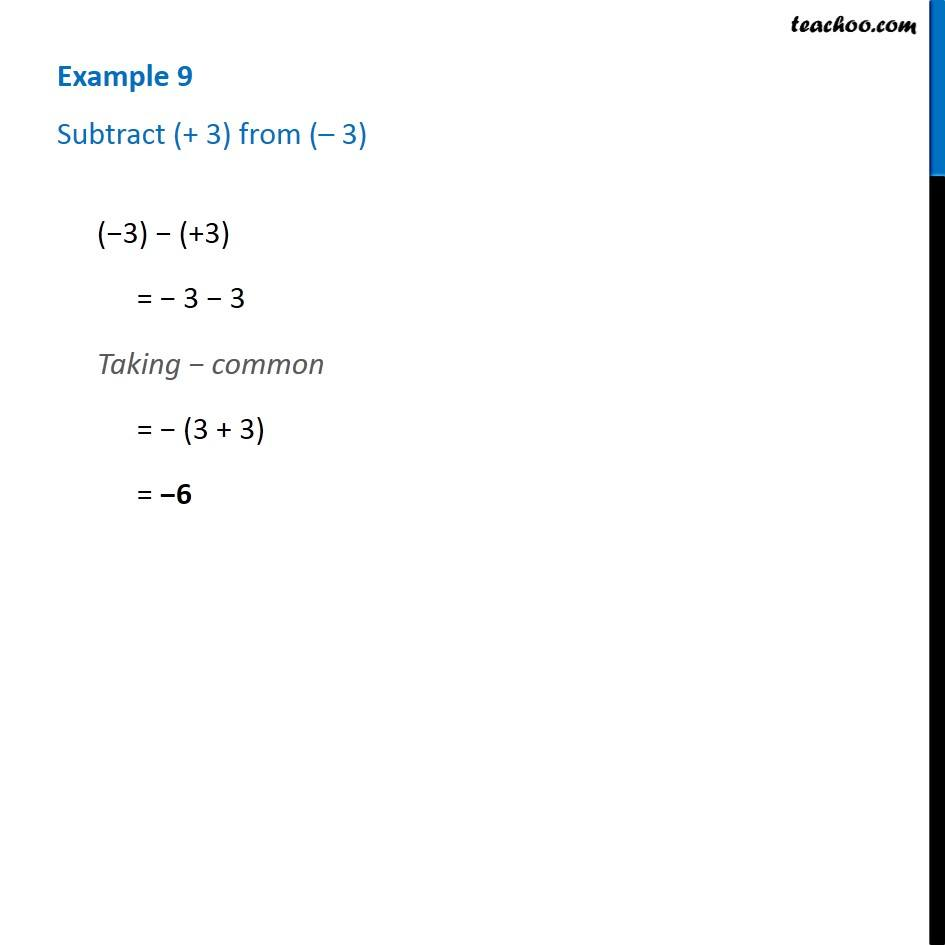1. Chapter 6 Class 6 Integers
2. Serial order wise
3. Examples

Transcript

Example 9 - Chapter 6 Class 6 Integers - NCERT Book Subtract (+3) from (-3) (-3) - (+3) = -3 - 3 Taking - common = -(3 + 3) = -6

Examples

Chapter 6 Class 6 Integers
Serial order wise